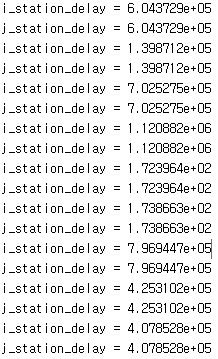# How to extract different values from the same array without duplicates?

7 ビュー (過去 30 日間)
youngwoo OH 2021 年 4 月 4 日
コメント済み: Rena Berman 2021 年 5 月 6 日 17:55
As a result of extracting using the datasample function from the state array with update information, all the same values were extracted.
I want to extract different update information.
....
for i = 1:1: user_num
% 3) delay update
state(i,3) = arrayfun(A, B); % (us),
% max_delay
max_t = max(state(i,3));
fprintf('max_t = %d\n', max_t );
% Returns 'i' observations uniformly randomly extracted from the delay index of the current cluster
i_station = datasample(state(i,3), 1); % Data of i-station in the same matrix
fprintf('i_station = %d\n', i_station);
j_station = datasample(state(i,3), 1); % Data of j-station in the same matrix
fprintf('j_station = %d\n', j_station);
% max| j_station - i_station|
at_t_delay = max(abs(j_station - i_station));
% delay 대소 비교에 따른 작업 조건
if (at_t_delay > max_t)
...
...
...
end
end
[Excuation result]##### 1 件のコメント表示非表示 なし
Rena Berman 2021 年 5 月 6 日 17:55

サインインしてコメントする。

### 採用された回答

Walter Roberson 2021 年 4 月 4 日
for i = 1:1: user_num
i is a scalar
state(i,3) = arrayfun(A, B); % (us),
3 is a scalar, and i is a scalar, so the left hand side represents a scalar. That can only work with the right hand side if the arrayfun() is outputing a scalar -- which would be an unusual use of arrayfun() but legal.
We cannot rule out the possibility yet that the arrayfun() is returning a scalar cell array, so there might be non-scalar data stored inside state(i,3)
max_t = max(state(i,3));
max() cannot be used on a cell array, so in order for the code to work here, state(i,3) must be numeric, in which case it must also be scalar, and max() of it will be the same as the value state(i,3)
i_station = datasample(state(i,3), 1); % Data of i-station in the same matrix
There is only one value in the scalar state(i,3) so datasample is going to return that one value.
j_station = datasample(state(i,3), 1); % Data of j-station in the same matrix
Still only one value in the scalar, so sampling is going to return the one value.
If you change the code so that you have a vector of values, say state(i,3,:) then
station_values = datasample(state(i,3,:), 2, 'replace', false);
i_station = station_values(1);
j_station = station_values(2);

サインインしてコメントする。

### Community Treasure Hunt

Find the treasures in MATLAB Central and discover how the community can help you!

Start Hunting!